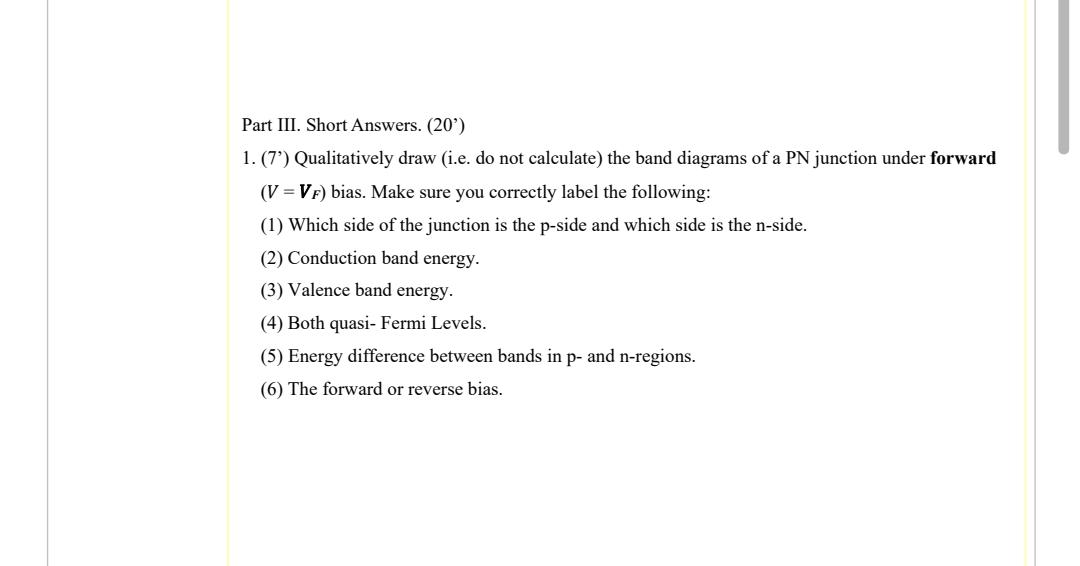# (Solved): Part III. Short Answers. (20') 1. (7') Qualitatively draw (i.e. do not calculate) the band diagram ...Part III. Short Answers. (20') 1. (7') Qualitatively draw (i.e. do not calculate) the band diagrams of a PN junction under forward $$\left(V=\boldsymbol{V}_{F}\right)$$ bias. Make sure you correctly label the following: (1) Which side of the junction is the p-side and which side is the $$\mathrm{n}$$-side. (2) Conduction band energy. (3) Valence band energy. (4) Both quasi- Fermi Levels. (5) Energy difference between bands in p-and n-regions. (6) The forward or reverse bias.

We have an Answer from Expert